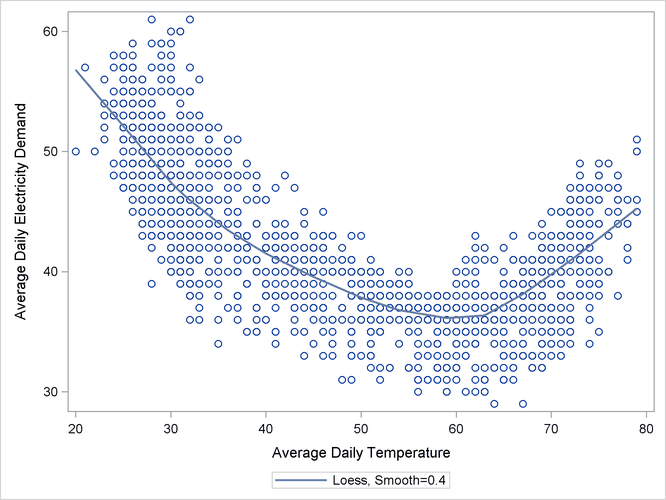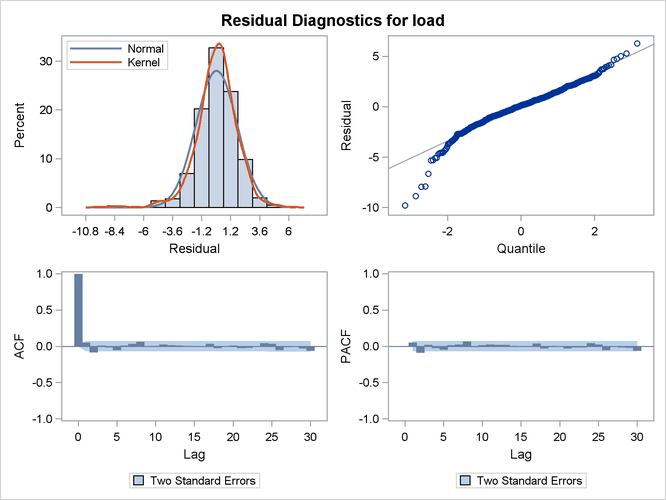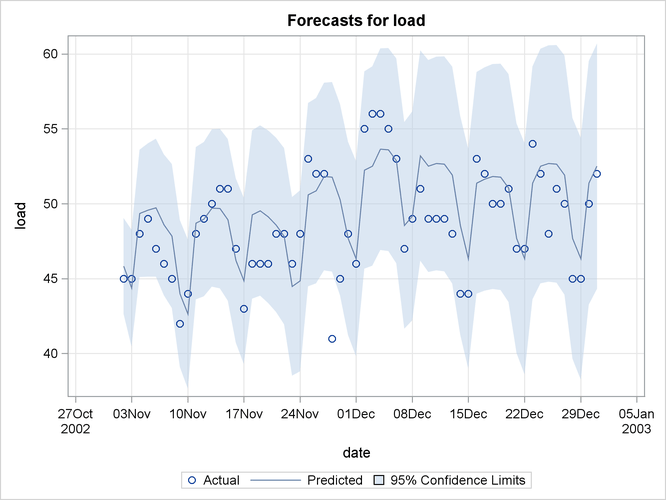The UCM Procedure

Example 34.6 Using Splines to Incorporate Nonlinear Effects

The data in this example are created to mirror the electricity demand and temperature data recorded at a utility company in the midwest region of the United States. The data set (not shown), `utility`, has three variables: `load`, `temp`, and `date`. The `load` column contains the daily electricity demand, the `temp` column has the average daily temperature readings, and the `date` column records the observation date.

The following statements produce a plot, shown in Output 34.6.1, of electricity load versus temperature. Clearly the relationship is smooth but nonlinear: the load generally increases when the temperatures are away from the comfortable sixties.

```proc sgplot data=utility;
run;
```

Output 34.6.1: Load versus Temperature PlotThe time series plot of the load (not shown) also shows that, apart from a day-of-the-week seasonal effect, there are no additional easily identifiable patterns in the series. The series has no apparent upward or downward trend. The following statements fit a UCM to the series that takes into account these observations. The particular choice of the model is a result of a little modeling exercise that compared a small number of competing models. The chosen model is adequate but by no means the best possible. The temperature effect is modeled by a deterministic three-degree spline with knots at 30, 40, 50, 60, and 75. The knot locations and the degree were chosen by visual inspection of the plot (Output 34.6.1). An autoreg component is used in place of the simple irregular component, which improved the residual analysis. The last 60 days of data are withheld for out-of-sample forecast evaluation (note the BACK= option in both the ESTIMATE and FORECAST statements). The OUTLIER statement is used to increase the number of outliers reported to 10. Since no CHECKBREAK option is used in the LEVEL statement, only the additive outliers are searched. In this example the use of the EXTRADIFFUSE= option in the ESTIMATE and FORECAST statements is useful for discarding some early one-step-ahead forecasts and residuals with large variance.

```proc ucm data=utility;
id date interval=day;
autoreg;
level plot=smooth;
splinereg temp knots=30 40 50 65 75 degree=3
variance=0 noest;
season length=7 var=0 noest;
estimate plot=panel back=60
outlier maxnum=10;
run;
```

The parameter estimates are given in Output 34.6.2, and the residual goodness-of-fit statistics are shown in Output 34.6.3. The residual diagnostic plots are shown in Output 34.6.4. The ACF and PACF plots appear satisfactory, but the normality plots, particularly the Q-Q plot, show possible violations. It appears that, at least in part, this nonNormal behavior of the residuals might be attributable to the outliers in the series. The outlier summary table, Output 34.6.5, shows the most likely outlying observations. Notice that most of these outliers are holidays, like July 4th, when the electricity load is lower than usual for that day of the week.

Output 34.6.2: Electricity Load: Parameter Estimates

The UCM Procedure

Final Estimates of the Free Parameters
Component Parameter Estimate Approx
Std Error
t Value Approx
Pr > |t|
Level Error Variance 0.21185 0.05025 4.22 <.0001
AutoReg Damping Factor 0.57522 0.03466 16.60 <.0001
AutoReg Error Variance 2.21057 0.20478 10.79 <.0001
temp Spline Coefficient_1 4.72502 1.93997 2.44 0.0149
temp Spline Coefficient_2 2.19116 1.71243 1.28 0.2007
temp Spline Coefficient_3 -7.14492 1.56805 -4.56 <.0001
temp Spline Coefficient_4 -11.39950 1.45098 -7.86 <.0001
temp Spline Coefficient_5 -16.38055 1.36977 -11.96 <.0001
temp Spline Coefficient_6 -18.76075 1.28898 -14.55 <.0001
temp Spline Coefficient_7 -8.04628 1.09017 -7.38 <.0001
temp Spline Coefficient_8 -2.30525 1.25102 -1.84 0.0654

Fit Statistics Based on Residuals
Mean Squared Error 2.90945
Root Mean Squared Error 1.70571
Mean Absolute Percentage Error 2.92586
Maximum Percent Error 14.96281
R-Square 0.92739
Random Walk R-Square 0.69618

Output 34.6.4: Electricity Load: Residual DiagnosticsObs Time Estimate StdErr ChiSq DF ProbChiSq
1281 04JUL2002 -7.99908 1.3417486 35.54 1 <.0001
916 04JUL2001 -6.55778 1.338431 24.01 1 <.0001
329 25NOV1999 -5.85047 1.3379735 19.12 1 <.0001
977 03SEP2001 -5.67254 1.3389138 17.95 1 <.0001
1341 02SEP2002 -5.49631 1.337843 16.88 1 <.0001
693 23NOV2000 -5.27968 1.3374368 15.58 1 <.0001
915 03JUL2001 5.06557 1.3375273 14.34 1 0.0002
1057 22NOV2001 -5.01550 1.3386184 14.04 1 0.0002
551 04JUL2000 -4.89965 1.3381557 13.41 1 0.0003
879 28MAY2001 -4.76135 1.3375349 12.67 1 0.0004

The plot of the load forecasts for the withheld data is shown in Output 34.6.6.

Output 34.6.6: Electricity Load: Forecast Evaluation of the Withheld Data